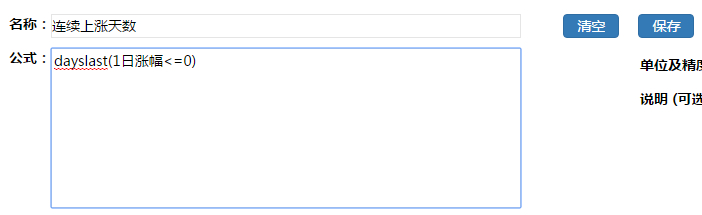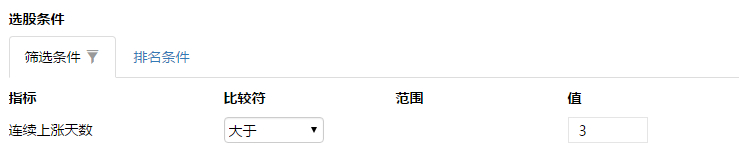1. 连续上涨天数 = “dayslast(1日涨幅<=0)”

2. 30日涨幅 = “后复权收盘价/ref(后复权收盘价，30)-1”

3. 30日累计换手率 = “Sum(当日换手率，30)”

4. 30日乖离率 = “后复权收盘价/MA(后复权收盘价,30)-1”

5. 30日波动率 = “stdev(1日涨幅,30)*sqrt(30)”

6. 五日均量比率 = “5日平均成交量/ref(5日平均成交量, 5)”

7. 今日昨日量比 = “当日成交量/ref(当日成交量, 1)”

8. 开盘涨幅 = “后复权开盘价/ref(后复权收盘价,1) - 1”

9. 开盘与前日最高价差 = “后复权开盘价 – ref(后复权最高价, 1)”

10. 创20日新高 = ”if (后复权最高价= max(后复权最高价, 20), 1, 0)“

11. 放量上攻 = " AND(今日昨日量比>2,1日涨幅>0.07)”

12. 10日收盘振幅 = " (max(后复权收盘价,10)-min(后复权收盘价,10)) /后复权收盘价"

13. 多头天数 = " Dayslast(And(5日复权均价 >20日复权均价, 20日复权均价 > 60日复权均价)<1)

14. 向上跳空两天不回补 = ” And(ref(后复权最低价,1) > ref(后复权最高价, 2) , 后复权最低价 > ref(后复权最高价, 2)) “

15. 上证指数成分股下跌比例 =“#bench.down.000001”

16. 个股相对沪深300涨幅 =" 1日涨幅 - #Bench.Change.000300”

17. 过去30天金叉次数 = "countdays(crossover(5日复权均价，20日复权均价), 30)“

18. 涨幅逐渐缩减

跌幅连续缩减天数>2
1日涨幅<0

19. 成本均价多头排列

20. 收盘价在5日成本均线上的个股数占总股票数的比例="countstock(收盘价>5日成本均线，0)/countstock(收盘价>0，0)"

21. 行业内缩量股占比="countstock(5日均量比例<0.9,1)/countstock(开盘价>0,1)"

22. 全市场当日最高换手率 = " Hmax(当日换手率,0) "

23. 低于行业内市盈率中值 = "市盈率<Hmed(市盈率，1)"

24. 布林线 = “ma(后复权收盘价,20)±stdev(后复权收盘价，20)*2

“+”为上线，“-”为下线﻿

25. 高于60日均线 = “后复权收盘价>60日复权均价

26.股票上市时的市值=“lastvalue(总市值,上市天数=1)

27.收长上影线=“And(Greater(后复权收盘价,后复权开盘价)/Less(后复权收盘价,后复权开盘价)>1.03,(后复权最高价-Greater(后复权收盘价,后复权开盘价))/ABS(后复权收盘价-后复权开盘价)>2)

28.MACD

DIF="EMA（后复权收盘价，12-EMA（后复权收盘价，26"

MACD="2*DIF-DEA"

29.RSI

RSI= "SMA(greater(后复权收盘价-昨日收盘价,0),14,1)/SMA(ABS(后复权收盘价-昨日收盘价),14,1)*100"

30.KDJ(9,3,3)

RSV = "(收盘价 - Min(最低价， 9)) / (max(最高价， 9) - min(最低价， 9)) * 100 "
K = "MA(RSV, 3) "
D = "MA(K, 3) "

J = "3*K- 2*D"

31.DPO(20,10,6)
DPO = 后复权收盘价-ref(ma(后复权收盘价,20),10)

32.MTM(12,6)

MTM:后复权收盘价-REF(后复权收盘价,12);

MAMTM:MA(MTM,6);

33.股票所在的行业市盈率

34. 日内分钟线函数

35. 月线MACD的实现

wDIF= EMA（  月收盘价， 12） - EMA（  月收盘价， 26）

wDEA = EMA（wDIF， 9）

36. 过去20天收盘价大于今天收盘价的天数 = 19-PercentRank(收盘价,20)*19

37. 过去20天里的第二高收盘价 =  percentile(收盘价， 0.95， 20)

38. 股票所属行业净利润增长 = hsum(归属于母公司所有者的净利润(TTM),1) / hsum(refQ(归属于母公司所有者的净利润(TTM), 4), 1) -1.